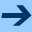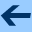Class Precedence List

# Class Precedence List

This section defines the algorithm for computing a class's linearized ancesters from its parents, its parent's parents, etc. GOO uses the C3 class linearization rule . The following is the GOO implementation of this algorithm:

```(dm class-ordered-ancestors (c|<class> => <lst>)
(def parents (class-parents c))
(rep merge-lists
((partial-cpl|<lst>
(lst c))
(remaining-lists|<lst>
(add (map class-ancestors parents) parents)))
(if (all? empty? remaining-lists)
(rev! partial-cpl)
(loc ((candidate (c)
(loc ((tail? (l|<lst>) (mem? (tail l) c)))
(and (not (any? tail? remaining-lists)) c)))
(candidate-at-head (l|<lst>)
(and (not (empty? l)) (candidate (head l)))))
(def next (any? candidate-at-head remaining-lists))
(if next
(loc ((del-next (l|<lst>)
(if (== (head l) next) (tail l) l)))
(merge-lists
(pair next partial-cpl)
(map del-next remaining-lists)))
(error "inconsistent precedence graph"))))))
```Class Precedence List